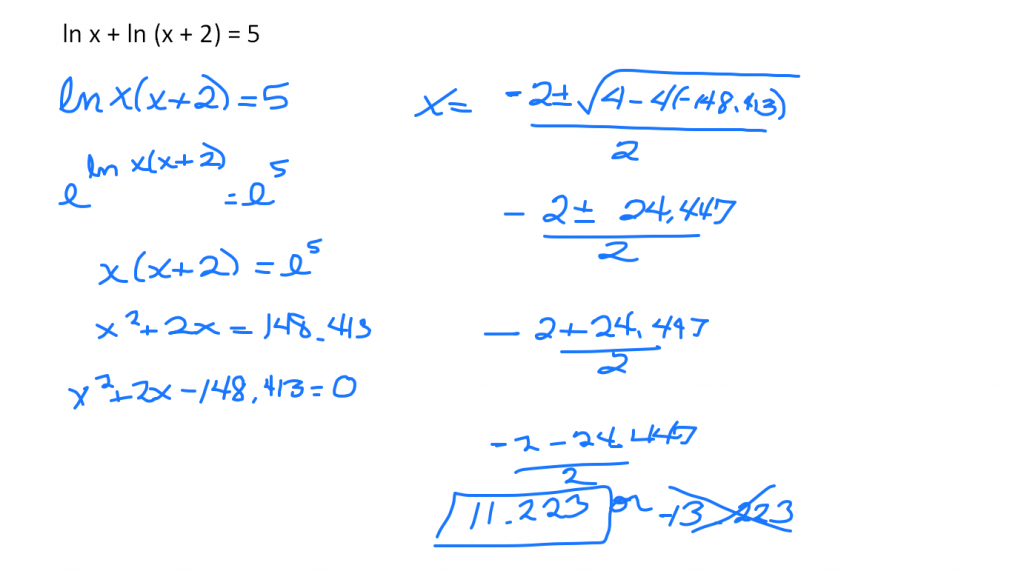# Exponential and Logarithmic Equations

//Exponential and Logarithmic Equations
Exponential and Logarithmic Equations 2017-11-13T22:01:53+00:00

Stacie Bender 9/21/14

## One to One Properties                           Inverse Properties

ax = ay if and only if x = y

logax = logay if and only if x = y

If the bases are the same, the exponents are equal.

If you need to remove a log­a, take a and raise it to both sides of the equation.

## Solve

Round answers to three decimal places.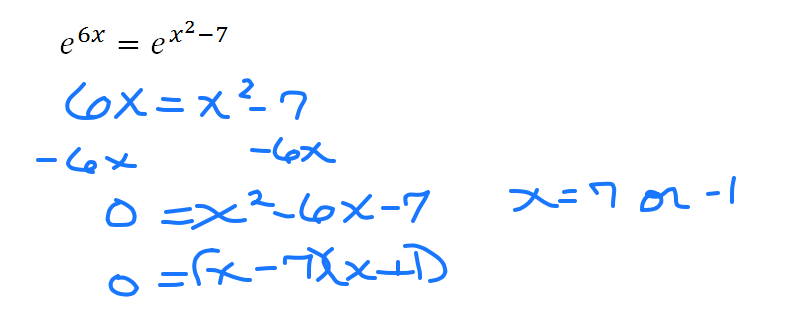2(5x + 3) = 128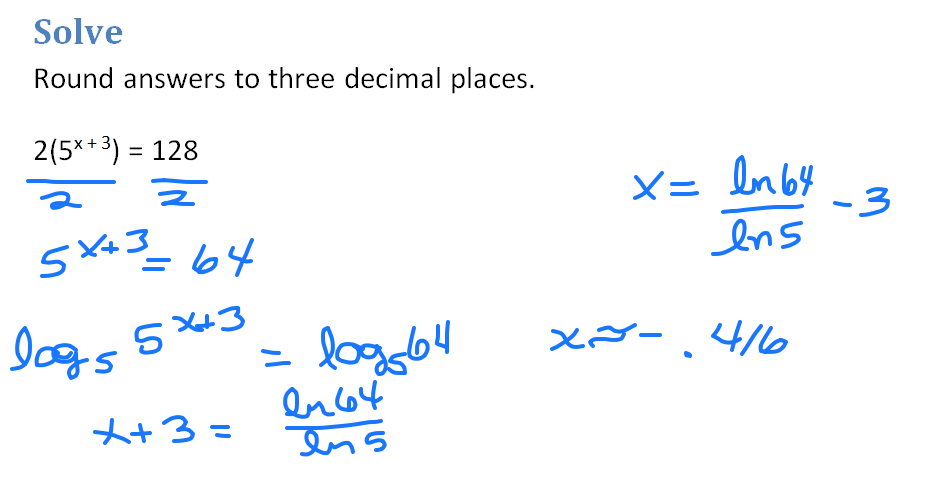2x + 1 = 16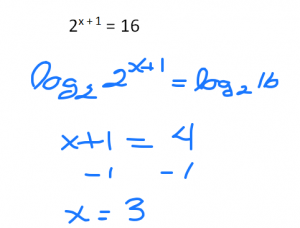e2x + 2ex – 8 = 0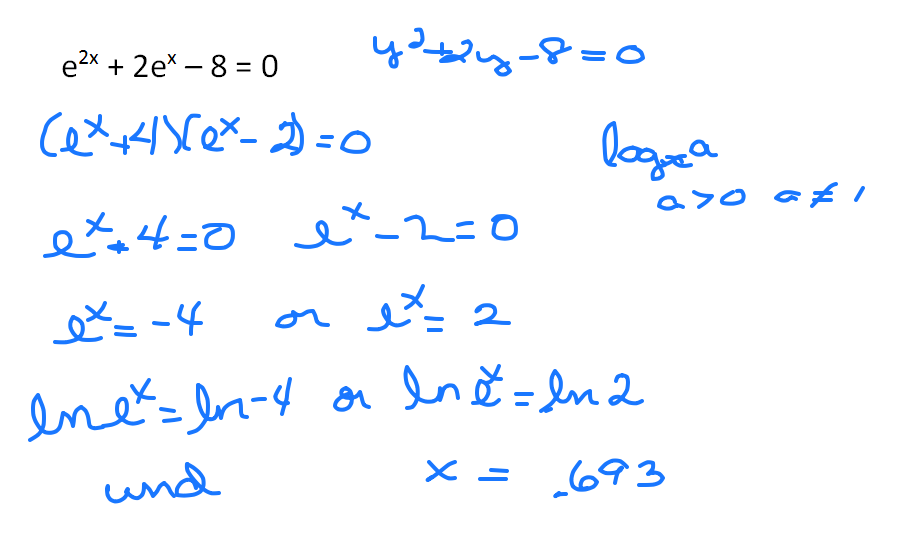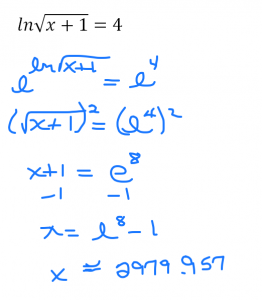ln x + ln (x + 2) = 5